# Differentiation of standard functions

## Summary and examples

In general the following derivatives of standard functions are assumed to be known.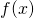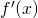a.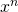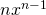b.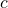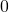c.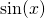d.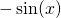e.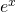f.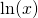g.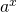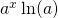h.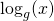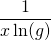Furthermore we have the following rules:

If:

i.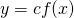then: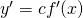and if:

j.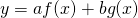then: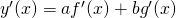In the following examples we show how these rules are applied.

##### Example 1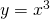so: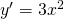(according to a)

##### Example 2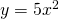so: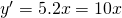(according to a, i)

##### Example 3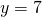so: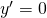(according to c)

##### Example 4so: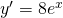(according to e, i)

##### Example 5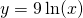so: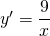(according to f, i)

##### Example 6so:(according to a, h, i, j)

##### Example 7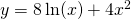so: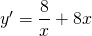(according to a, f, i, j)

Now some more difficult examples.

##### Example 8

Find the derivative of: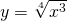At first sight this function is not in the table of standard functions. However we can rewrite the function: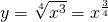and thus rule a. can be applied with: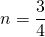So: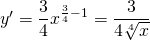##### Example 9

Differentiate: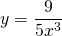This function can be written as: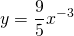and thus: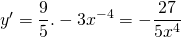(according to a,i)

##### Example 10

A difficult function seems: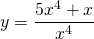but also this function can be rewritten and be differentiated easily: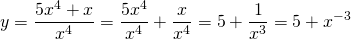and thus: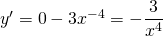(according to a, b, j)

##### Example 11

Differentiate the following function: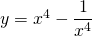We can rewrite the function as follows:and thus: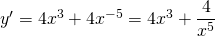(according to a, i)

##### Example 12

Finally we want to differentiate the following function: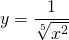We can rewrite it as follows: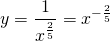The derivative is: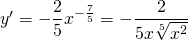0